Takes an image and applys a convolution operation to it, using a user-supplied or built-in kernel. This function uses a fast-fourier transform and does the convolution in the frequency domain, so it should be faster for much larger kernels.

render_convolution_fft(
image,
kernel = "gaussian",
kernel_dim = c(11, 11),
kernel_extent = 3,
absolute = TRUE,
filename = NULL,
preview = FALSE,
gamma_correction = FALSE
)

## Arguments

image

Image filename or 3-layer RGB array.

kernel

Default gaussian. By default, an 11x11 Gaussian kernel with a mean of 0 and a standard deviation of 1, running from -kernel_extent to kernel_extent. If numeric, this will be the standard deviation of the normal distribution. If a matrix, it will be used directly as the convolution kernel (but resized always to be an odd number of columns and rows).

kernel_dim

Default c(11, 11). The dimension of the gaussian kernel. Ignored if user specifies their own kernel.

kernel_extent

Default 3. Extent over which to calculate the kernel.

absolute

Default TRUE. Whether to take the absolute value of the convolution.

Default 50. Amount to pad the image to remove edge effects.

filename

Default NULL. The filename of the image to be saved. If this is not given, the image will be plotted instead.

preview

Default FALSE. Whether to plot the convolved image, or just to return the values.

gamma_correction

Default FALSE. Controls gamma correction when adding colors. Default exponent of 2.2.

## Value

3-layer RGB array of the processed image.

## Examples

#if(interactive()){
#Perform a convolution with the default gaussian kernel

plot_image(dragon)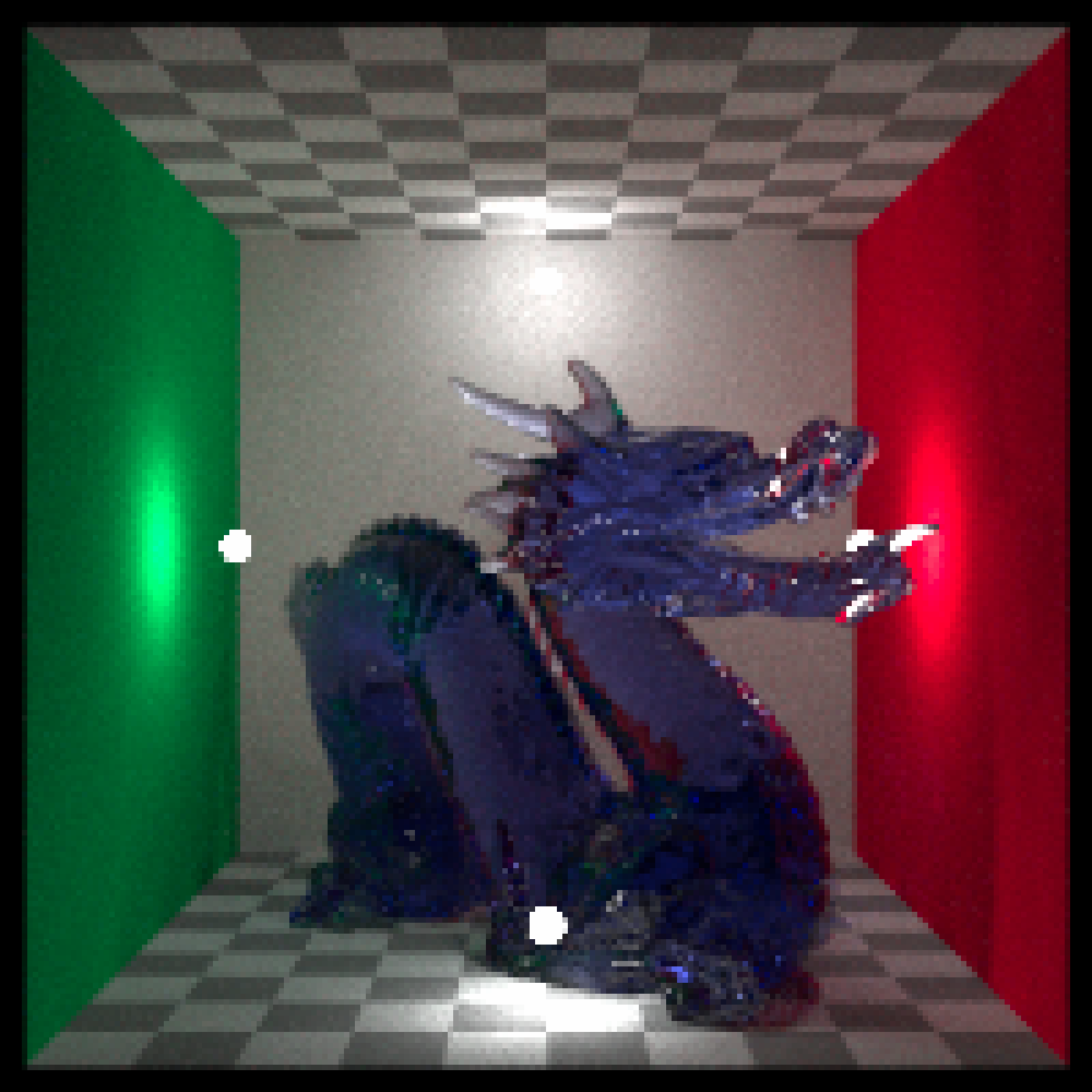#Perform a convolution with the default gaussian kernel
render_convolution_fft(dragon, kernel=0.1,preview = TRUE)#Increase the width of the kernel
# \donttest{
render_convolution_fft(dragon, kernel = 2, kernel_dim=21,kernel_extent=6, preview = TRUE)# }

#Use a built-in kernel:
# \donttest{
render_convolution_fft(dragon, kernel = generate_2d_exponential(falloff=2, dim=31, width=21),
preview = TRUE)# }
#Perform edge detection
# \donttest{
edge = matrix(c(-1,-1,-1,-1,8,-1,-1,-1,-1),3,3)
render_convolution_fft(dragon, kernel = edge, preview = TRUE)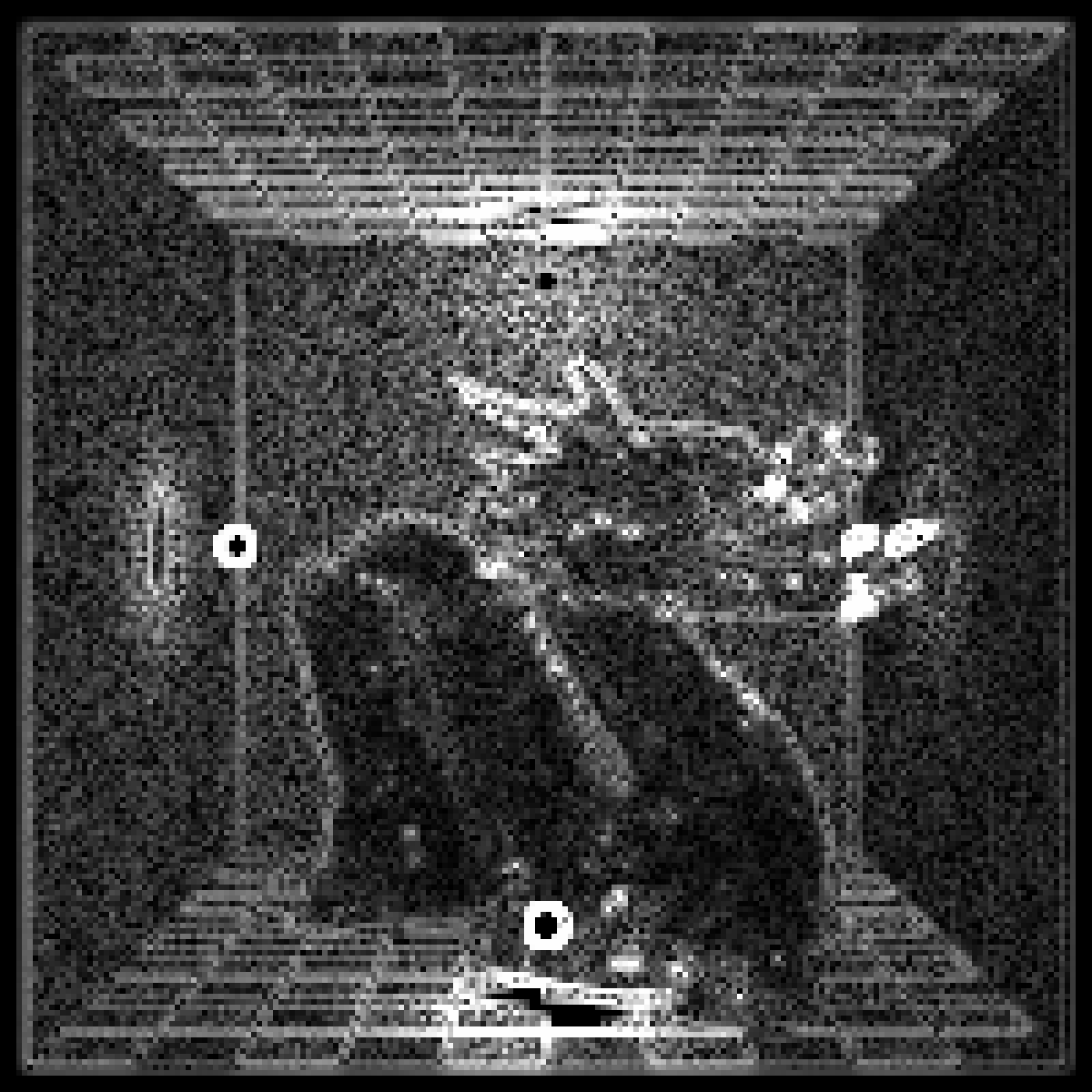# }

#Perform edge detection with Sobel matrices
# \donttest{
sobel1 = matrix(c(1,2,1,0,0,0,-1,-2,-1),3,3)
sobel2 = matrix(c(1,2,1,0,0,0,-1,-2,-1),3,3,byrow=TRUE)
sob1 = render_convolution_fft(dragon, kernel = sobel1)
sob2 = render_convolution_fft(dragon, kernel = sobel2)
sob_all = sob1 + sob2
plot_image(sob_all)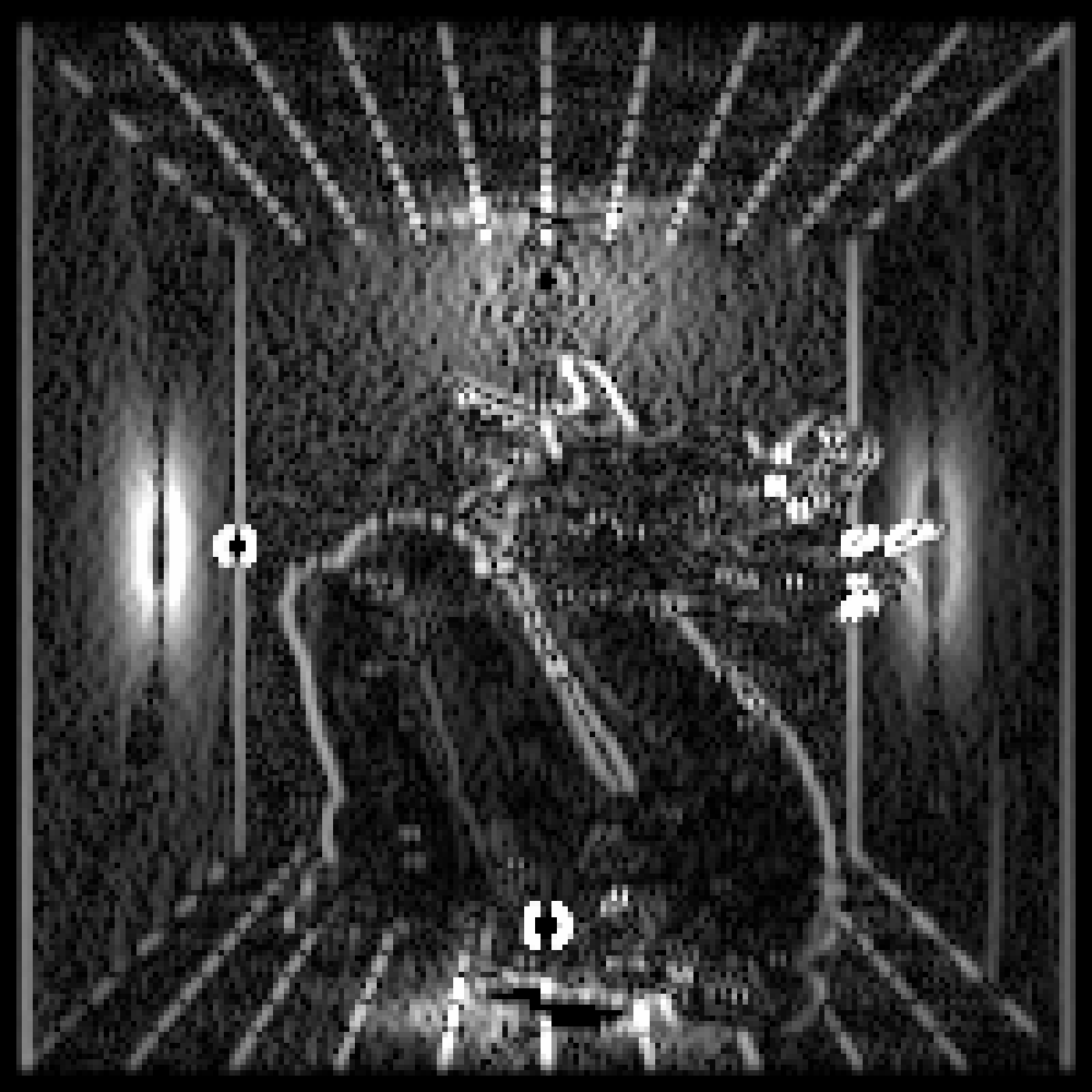# }

#We can also apply this function to matrices:
# \donttest{
volcano %>% image()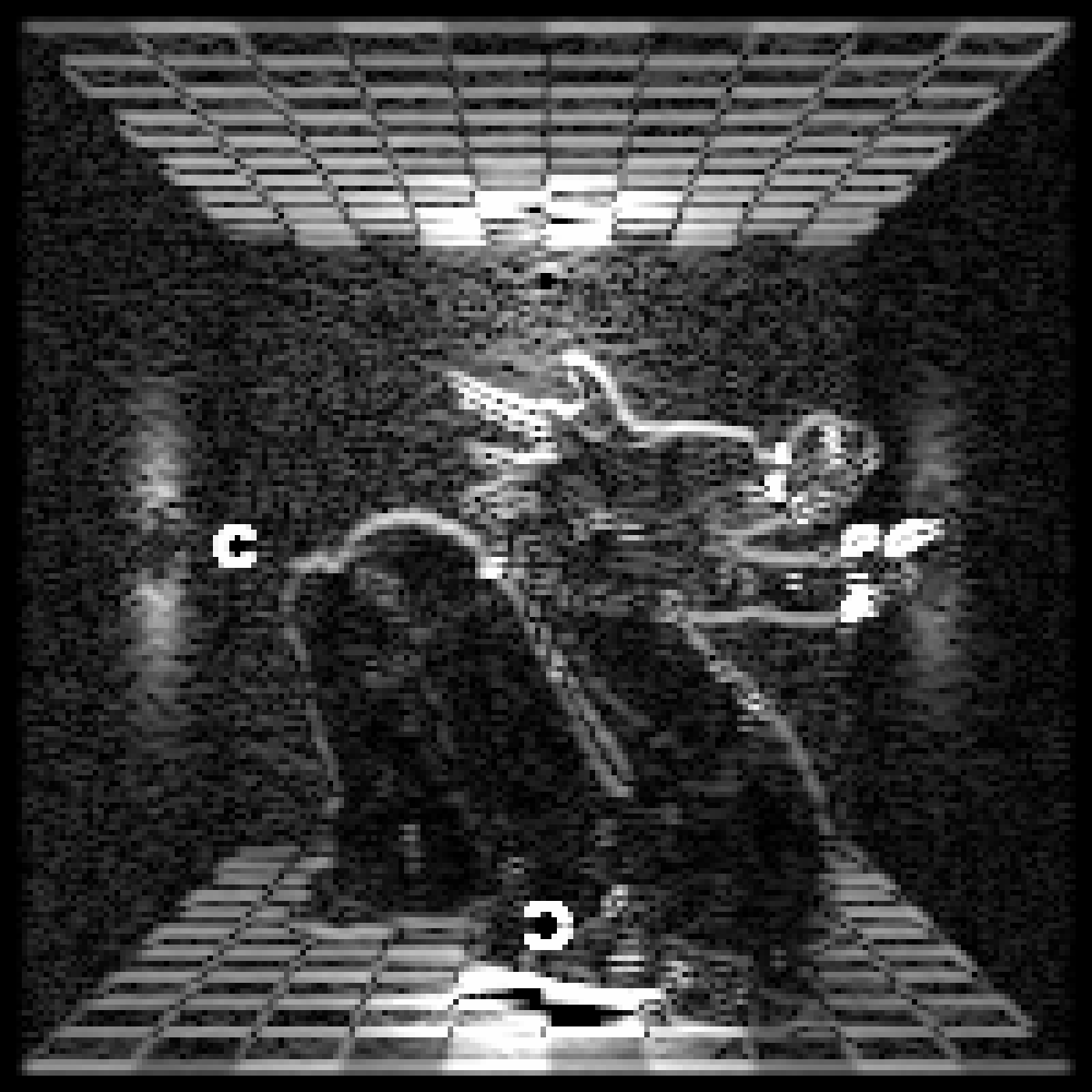volcano %>%
render_convolution_fft(kernel=generate_2d_gaussian(sd=1,dim=31)) %>%
image()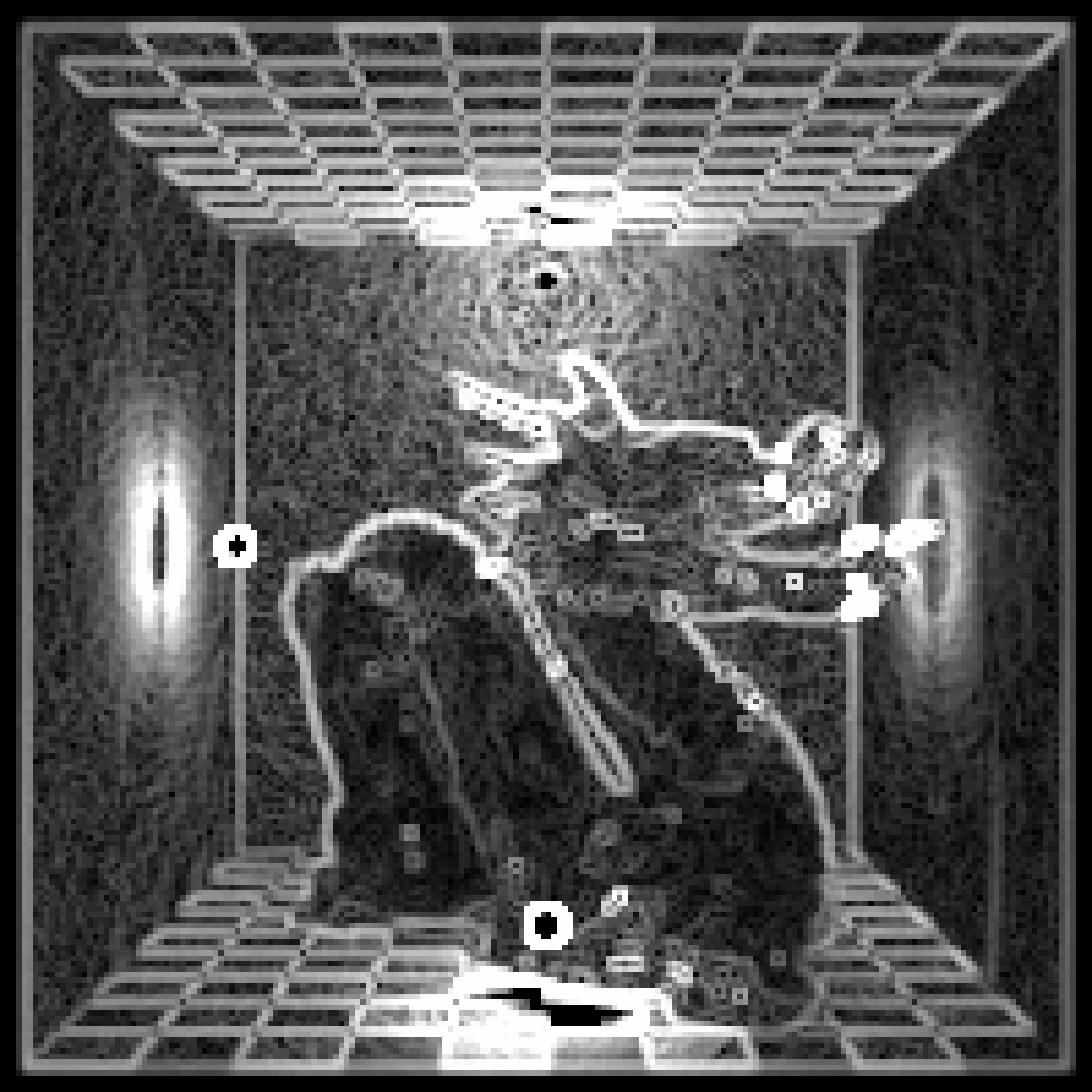# }
#Because this function uses the fast-fourier transform, large kernels will be much faster.
# \donttest{
render_convolution_fft(dragon, kernel = , preview = TRUE)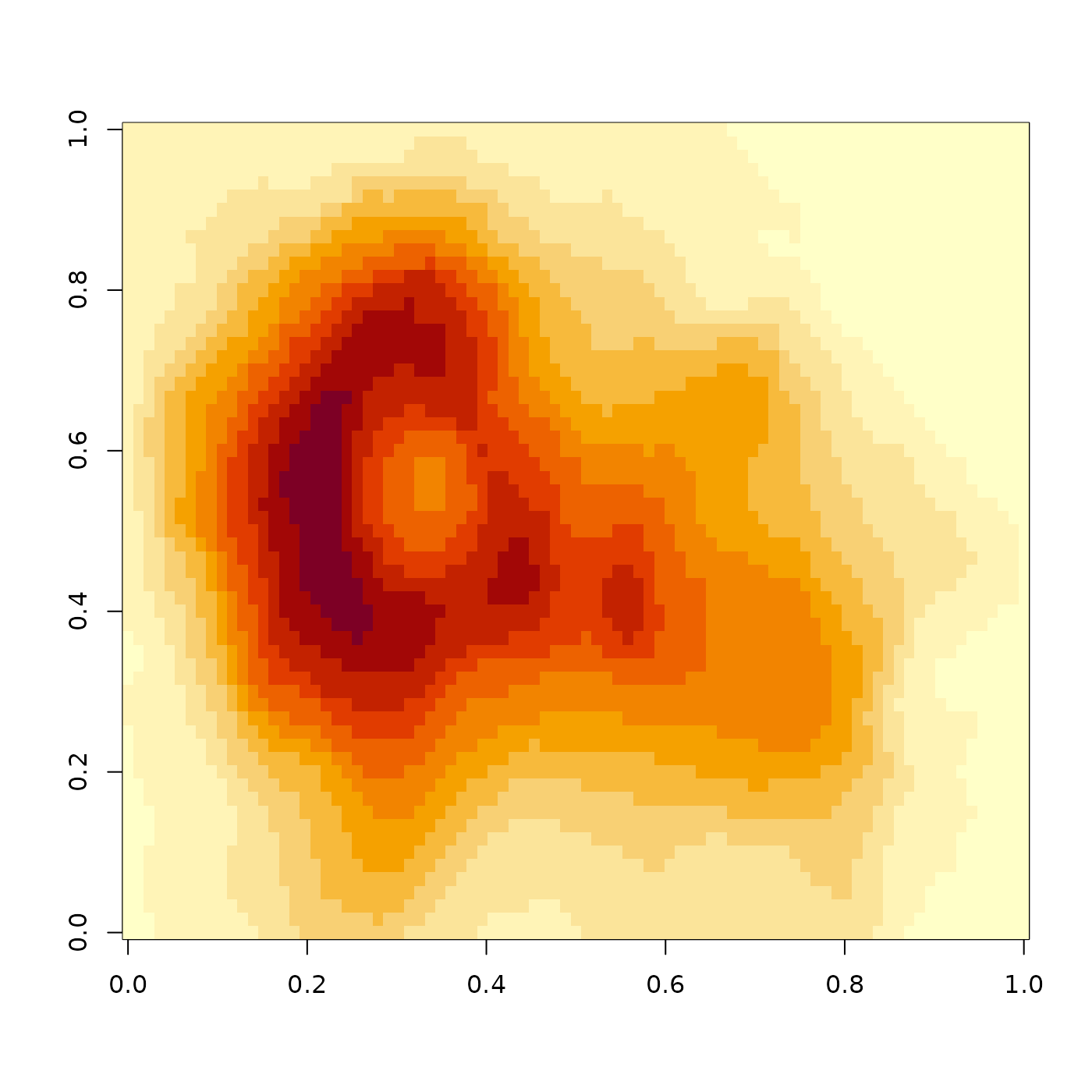# }

#Use a custom kernel (in this case, an X shape):
custom = diag(10) + (diag(10)[,10:1])
#Normalize
custom = custom / 20
# \donttest{
plot_image(custom*20)
#> Error in plot_image(custom * 20): object 'xlim' not found
render_convolution_fft(dragon, kernel = custom, preview = TRUE)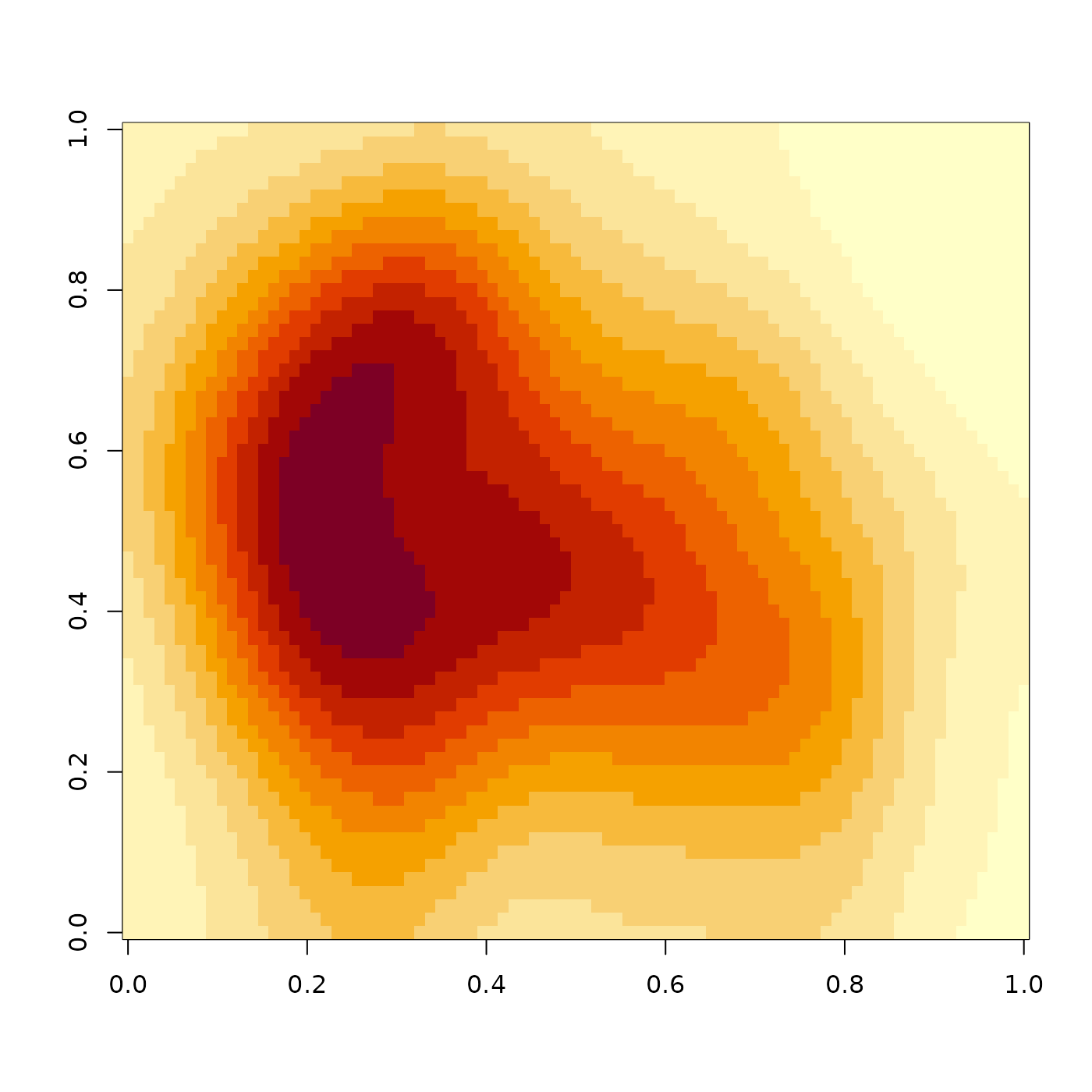# }
#end}